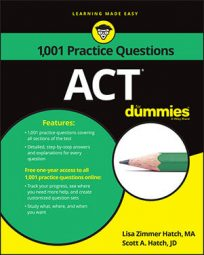##### ACTNot only is factoring fun, but it's also a skill that will earn you valuable points on the ACT Math exam. Try out these practice questions, where you have to factor a quadratic expression and find the value of x in a quadratic equation.

## Practice questions

1. Which of the following is a factor of a2 – 14a – 15?

A. a + 5 B. a + 3 C. a – 1 D. a – 3 E. a – 15

2. For what values of x does x4 – 20x2 – 64 = 0?

A. 16 and –4 only B. –16 and 4 only C. 1, 4, –1, and –4 only D. 4 only E. 4, 2, –4, and –2 only

1. The correct answer is Choice (E).

The easiest way to approach this problem is to first consider the last term in the expression, the –15. What are the factors of –15 that sum to –14? They could only be (a – 15) and (a + 1). When you multiply (a – 15)(a + 1), you get the desired quadratic, a2 – 14a – 15. Because a + 1 isn't an option, the answer has to be Choice (E).

2. The correct answer is Choice (E).

When you see a quadratic equation, your first thought should be to find its binomial factors. When you factor, you'll likely discover the next step.

Find the square root of the first term: x2. Then consider the last term of –64 and ask yourself what factors of –64 have a sum of –20. Those two factors are –16 and –4, so the binomial factors of the quadratic are (x2 – 16)(x2 – 4).

At this point, you may be tempted to pick Choice (A), but you aren't through; you can factor the terms further. Notice that the binomial factors are the difference of perfect squares. Finding their factors is easy. The two factors are the sum and difference of the square roots of each perfect square in the expression. So when you factor (x2 – 16), you get (x + 4)(x – 4). When you factor (x2 – 4), you get (x + 2)(x – 2). The fully factored quadratic is (x + 4)( x – 4) (x + 2)(x – 2) = 0. The expression in its entirety equals 0 when any one of these factors equals 0. Set each equal to 0, and you see that the full set of values for x that solve the equation are 4, 2, –4, and –2, which is Choice (E).